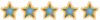# Anthing i need to change##Anthing i need to change

hi guys is there anything i need to change in my profile/settings to make my bot call ie my bot says call upto but never dose, its just folds

************** SETTINGS *************

[Poker]
loop_pause = 1000
nopopupcheck = 0

[Player]
player_file = BetaBot
player_chat = 0

[NETWORK]
yahoo = 14540253

[Table]
table_min_players = 5
table_max_players = 8
table_min_blind = 2K
table_max_blind = 4K

[time_servers]
0000-0059 = Random
0100-0159 = Random
0200-0259 = Random
0300-0359 = Random
0400-0459 = Random
0500-0559 = Random
0600-0659 = Random
0700-0759 = Random
0800-0859 = Random
0900-0959 = Random
1000-1059 = Random
1100-1159 = Random
1200-1259 = Random
1300-1359 = Random
1400-1459 = Random
1500-1559 = Random
1600-1659 = Random
1700-1759 = Random
1800-1859 = Random
1900-1959 = Random
2000-2059 = Random
2100-2159 = Random
2200-2259 = Random
2300-2359 = Random

[avoid_servers]
1 = Ankara
2 = California
3 = Istanbul
4 = Izmir
5 = Macau
6 = Istanbul
7 = Bursa
8 = Antalya
9 = Mesa

[Log]
data_path = data
log_path = log
log_text = 1
delete_old_txt = 1
log_hand = 1
log_server = 0
delete_old_logs = 0
log_screen_blind = 1
log_screen_action = 1
log_screen_card = 1
log_screen_capture = 1

[Browser]
browser_file = chrome.exe

[BotDetection]
pauseafter = 2400
resumeafter = 10

[ERRORS]
stop on none fatal errors = 0

[RaiseBox]
raisebox = 0

[Usage]
usage_send = 0

user = bot

[Gui_Position]
x = 1095
y = 5

[Resize]
chips = 5
raise = 5

[NewRoom]
high = 25

[ForceNewRoom]
forceserver = 200
hands = 30
staylonger = 5

[ForceBank]
low = 15

[ForcePause]
DownPercent = 10

[popup_check]
rounds = 2

[auto_blind]
on = 1

[set_opponents]
score = 3

[ROOMS]
500M = 3555095780
100M = 601454552
40M = 1022196536
20M = 3422649495
10M = 1595511023
4M = 3047067494
2M = 3216870985
1M = 2165606653
800k = 2088473034
400k = 2653132110
200k = 907056831
80k = 2512950585
40k = 2236847123
20k = 1990106390
10k = 4112752692
4k = 1560126419
2k = 4204569927
1k = 981705653
400 = 2005771633
200 = 4110917621
50 = 1580180246
20 = 2836899515
10 = 953198639
4 = 2955516681
2 = 2398460381

[PLAYERS]
9 = 953852009
8 = 300327035
7 = 3670455426
6 = 2737610920
5 = 3747784777
4 = 3076172824
3 = 981052710
2 = 1787537358
1 = 3224353503
0 = 754033921

[CONFIG]
skipfirstserverchange = 1
selectrandomserver = 0
sethighpriority = 1
holdemcksm = 451734754
lobbycolour = 134436
lobbychecksum = 957810222
scrolllopixel = 0x94999B
scrollhipixel = 0xA1A6A7
fullcksm = 1689135299
emptycksm = 2059614732
tablecolour = 658210
tablechecksum = 958989874
tolobby = 2605626683
buddy = 2235952
tblstndg = 382872467

[TWEAKS]
findroomspeed = 1
playturnspeed = 1
mousespeed = 1
playfasttables = 0

************** PROFILE *************

[Non_Suited_Preflop_Hands]
all_in = AA KK QQ JJ TT AK
raise_strong =
raise =
call_strong = AQ
call_any =
call_upto_high = 99 88 AJ AT KQ KJ KT
call_upto_low = 77 66 55 44 33 22 A9 A8 A7 A6 A5 A4 A3 A2 QJ QT
call_once =
small_blind =

[Suited_Preflop_Hands]
all_in = AK
raise_strong = AQ AJ
raise =
call_strong =
call_any =
call_upto_high = AT KQ KJ KT
call_upto_low = A9 A8 A7 A6 A5 A4 A3 A2 K9 QJ QT JT
call_once =
small_blind =

[call_upto_high]
preflopcallamount = 4
flopcallamount = 4
turncallamount = 8
rivercallamount = 8

[call_upto_low]
preflopcallamount = 2
flopcallamount = 2
turncallamount = 4
rivercallamount = 4

[raise_strong]
preflopraiseamount = 5
flopraiseamount = 10
turnraiseamount = 10
riverraiseamount = 20

[raise]
preflopraiseamount = 10
flopraiseamount = 5
turnraiseamount = 10
riverraiseamount = 15

[FlopScore]
0-20 = check
21-40 = call_upto_low
41-55 = call_upto_high
56-60 = call
61-65 = call
66-75 = call
76-200 = raise_strong

[TurnScore]
0-25 = check
26-45 = call_upto_low
46-65 = call_upto_high
66-70 = call
71-80 = call
81-200 = all_in

[RiverScore]
0-30 = check
31-50 = call_upto_low
51-65 = call_upto_high
66-70 = call
71-200 = all_in

************** REPORT **************

OS: WIN_VISTA
Arch: X86
Screen Res: 1280 x 800
Depth: 32
Memory: 2048 MB
DPI: 96
Cores/Processors: 1
ZPB Version: 2011.1.4.6
Flashplayer version: 11.6.602.180
Using Browser: Chrome 25.0.1364.172

plz help me

Posts : 17
Join date : 2013-03-23
Location : Scotland

##Re: Anthing i need to change

Did you complete step 3 correctly ?AndyboyPosts : 306
Join date : 2012-03-24
Age : 42
Location : UK

##Re: Anthing i need to change

yea i had re-do step 3 but i got it done right

Posts : 17
Join date : 2013-03-23
Location : Scotland

##Re: Anthing i need to change

just had a pair of 9 and it folded, it saved me but not the point

Posts : 17
Join date : 2013-03-23
Location : Scotland

##Re: Anthing i need to change

Ok i notice your pc only has 1 processor what speed does it run at ?

When you say your bot is folding all the time is it that, or is it timing out ?AndyboyPosts : 306
Join date : 2012-03-24
Age : 42
Location : UK

##Re: Anthing i need to change

No I runs fine, only folds on calls up to and calls and down to, it dose call for time to time and it gose as all in to, but no prob with time out or processor

Posts : 17
Join date : 2013-03-23
Location : Scotland

##Re: Anthing i need to change